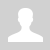Python. Lists. Functions and methods

11.2.3.3 apply functions and methods of processing lists

Python. Lists. Functions and methods

A list is an ordered composite data type, a mutable collection of items of different types.

Functions/methods of lists.

Notes:

lisls: lst, lst1, lst2, nums
element of list: x
index: i
interger: n

 Function/Method Example Describtion x in lst lst = [2, 3, 4] print(3 in lst) # True print(5 in lst) # False Checking that x is contained in lst x not in lst print(3 not in [2, 3, 4]) # False print(5 not in [2, 3, 4]) # True Checking that x is not contained in lst lst1 + lst2 lst1 += lst2 lst1 = [1, 2] lst2 = [3, 4] print(lst1 + lst2) # [1, 2, 3, 4] Concatenation of lists. A new list that contains first the elements lst1 and then all the elements lst2 lst * n lst *= n lst = [2, 3] print(lst * 3) # [2, 3, 2, 3, 2, 3] List lst repeated n times lst[i] lst = ['A', 'B', 'C', 'D'] print(lst) # B print(lst[-2]) # C i-th element of the list, negative i - for counting from the end lst[start:stop:step] lst = ['A', 'B', 'C', 'D'] print(lst[1:3]) # ['B', 'C'] print(lst[::2]) # ['A', 'C'] Slice of the list len(lst) lst = ['A', 'B', 'C', 'D'] print(len(lst)) # 4 List length max(lst) nums = [9, 7, 4, 6] print(max(nums)) # 9 Maximum list item min(lst) nums = [9, 7, 4, 6] print(min(nums))  # 4 Minimum list item sum(lst) nums = [9, 7, 4, 6] print(sum(nums))  # 26 Sum of list items lst.index(x) nums = [9, 7, 4, 6] print(nums.index(4))  # 2 Index of the first occurrence of x in lst (give an error if x is not in lst) lst.count(x) nums = [7, 7, 4, 6, 7] print(nums.count(7))  # 3 The number of occurrences of x in lst lst.append(x)     lst.insert(i, x) nums = [2, 7, 4] nums.append(6) print(nums)  # [2, 7, 4, 6] nums = [2, 7, 4] nums.insert(1, 6) print(nums)  # [2, 6, 7, 4] nums.insert(10, 3) print(nums) # [2, 6, 7, 4, 3] Add x to the end of lst   Insert x to the lst[i] position. Elements to the right of insertion change index to i + 1 lst1.extend(lst2) lst1 = [2, 7] lst2 = [4, 6] lst1.extend(lst2) print(lst1) # [2, 7, 4, 6] Add elements of collection lst2 to the end of lst1 del lst[i] nums = [9, 7, 4, 6] del nums print(nums) # [9, 4, 6] Remove the i-th element of the list del lst[start:stop:step] nums = [9, 7, 4, 6] del nums[:2] print(nums) # [4, 6] Remove all elements from lst that are in the slice lst.clear() nums = [9, 7, 4, 6] nums.clear() print(nums) # [] Remove all elements from lst (same as del lst[:]) lst.copy() nums = [9, 7, 4, 6] lst = nums.copy() print(lst) # [9, 7, 4, 6] Copy of list (same as lst[:]) lst.remove(x) nums = [9, 7, 4, 6] nums.remove(7) print(nums) # [9, 4, 6] Delete the first occurrence of x in lst, in case x not in lst - an error lst.pop(i) nums = [9, 7, 4, 6] nums.pop(2) print(nums) # [9, 7, 6] nums.pop() print(nums) # [9, 7] Get the ith element of the list and remove it from the list at the same time. With no arguments, removes the last element: a.pop () == a.pop (-1) lst.reverse() nums = [9, 7, 4, 6] nums.reverse() print(nums) # [6, 4, 7, 9] Reverse the order of elements in a (reverse the list) lst.sort() nums = [9, 7, 4, 6] nums.sort() print(nums) # [4, 6, 7, 9] Sort the list in ascending order lst.sort(reverse=True) nums = [9, 7, 4, 6] nums.sort(reverse=True) print(nums) # [9, 7, 6, 4] Sort the list in descending order bool(lst) lst = ['apple'] print(bool(lst)) # True lst.pop() print(bool(lst)) # False Checking a list for emptiness

Questions:

1. What is the data structure 'list'?
2. Provide features of the data structure 'list'?
3. Name three functions of the data structure 'list'.
4. Name three methods of the data structure 'list'.

Exercises:

Ex.1 Identify result of outputs (Designed by Mr. Halil Mali - CS teacher of NIS Uralsk)

Ex.2 Identify result of outputs (Designed by Mr. Halil Mali - CS teacher of NIS Uralsk)

Ex.3 Identify result of outputs (Designed by Mr. Halil Mali - CS teacher of NIS Uralsk)

Ex.4 Identify result of outputs (Designed by Mr. Halil Mali - CS teacher of NIS Uralsk)